# The Art Of Computer And Mathematics: Barnsley Fern

Engineeringby Sabin

It is funny to see Art, Computer and Mathematics on the same line !! but some weird combinations can create awesome results. In this article we will be looking at how we can code an algorithm that draws a Fern.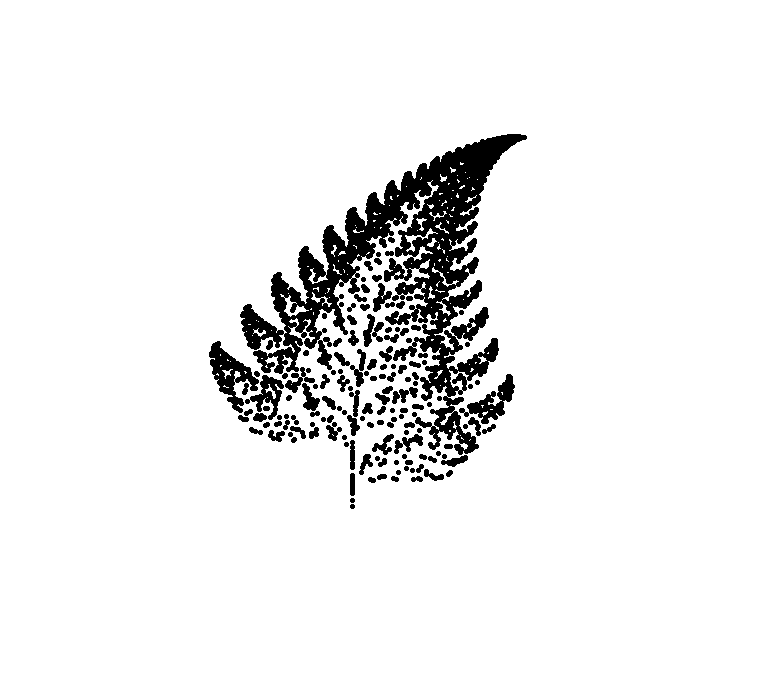Barns ley Fern

Yehh!! You read it right we are going to draw a fern with numbers. I was so fascinated with the idea that we could create an art with simple mathematical algorithms . I always thought mathematics in computer science were boring.

If you are interested on, What more can be created using mathematical algorithms then check this link

So there are couple of implementation for this algorithm a more simpler approach can be found here.

Since I wanna flex my shit code writing skills, I will follow slightly more complex approach i.e using only matrix for computation. A quick backdrop on prerequisites for my version of implementation.

1. python
2. numpy
3. turtle
4. pyscreenshot

Lets Get Started

As I mentioned earlier we will be implementing it using matrices.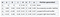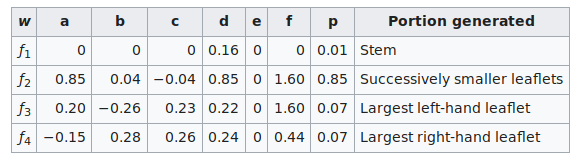These are the values responsible for drawing individual part of a fern. Its more like a affine transformation on some coordinates. All we do is multiply coordinates with some per-defined matrices.

The intuition behind this algorithm is that we generate a random probability which in this case is a random number that will be responsible for drawing different parts of fern. For example if the randomly generated probability is less than 0.01 the algorithm will draw steam and so on.

You can find the complete source code at : Click . Here I will be doing line by line explanation of source codes .

Explanation:

Unlike any other script first we import the libraries required

``````import turtle
import numpy as np
import random
import pyscreenshot
``````

So in my version of implementation we will be creating a class which can be of any name but I settled on DrawFern

``````class DrawFern:
``````

In __init__ function it take 4 parameters each to make our script more customizable which I think are self explanatory.

``````self.stem = np.array([[0, 0], [0, 0.16]])
self.stem_c = np.array([, ])
self.smaller_leaflets = np.array([[0.85, 0.04], [-0.04, 0.85]])
self.smaller_leaflets_c = np.array([, [1.60]])
self.left_large_leaflets = np.array([[0.20, -0.26], [0.23, 0.22]])
self.left_leaflets_c = np.array([, [1.6]])
self.right_large_leaflets = np.array([[-0.15, 0.28], [0.26, 0.24]])
self.right_large_leaf_c = np.array([, [0.44]])
``````

Each of these line represents a matrix containing values as defined in the table mentioned above. [ Note _c references coefficient ]

``````def generate_points(self, matrix, coefficient):
if self.show_points:
print(f'Drawing Point At: {self.x_y}')
``````

Next what we did was we created a function namely generate_points() which takes a matrix and coefficient as an input then performs matrix multiplication on x,y coordinates and adds coefficient. Then the output of these operation is set as our new x and y coordinates.

``````for _ in range(self.loop_over):
self.plot_point()
``````

Similarly in our start function we iterate over self.loop_over times. And on each iteration we begin with plotting previously calculated coordinates then generate a random number.

``````random_number = random.random() * 100
``````

You can multiply it with 100 to scale it up by 100 times or just play with the generated number. I prefer scaling it up.

``````if random_number < 1:
self.generate_points(self.stem, self.stem_c)
elif random_number < 86:
self.generate_points(self.smaller_leaflets, self.smaller_leaflets_c)
elif random_number < 93:
self.generate_points(self.left_large_leaflets, self.left_leaflets_c)
else:
self.generate_points(self.right_large_leaflets, self.right_large_leaf_c)
``````

At last all we do is call the respective function to draw different parts of the fern. For an example if the probability is less than 0.001, Then we draw stem of the fern similarly respective parts are drawn according to the random probability generated.

OUTPUT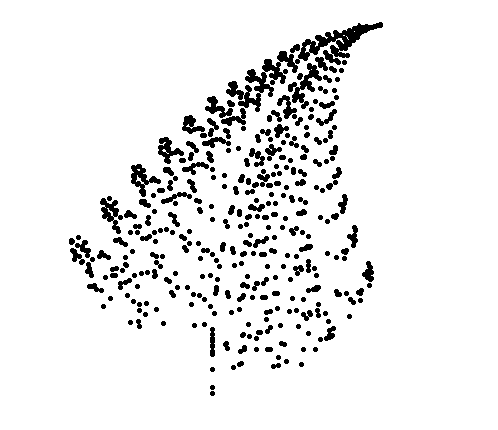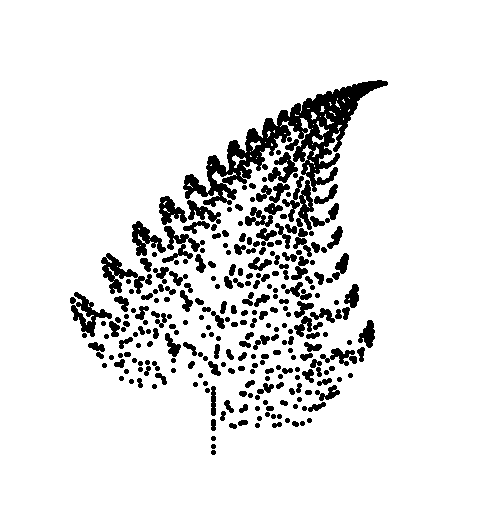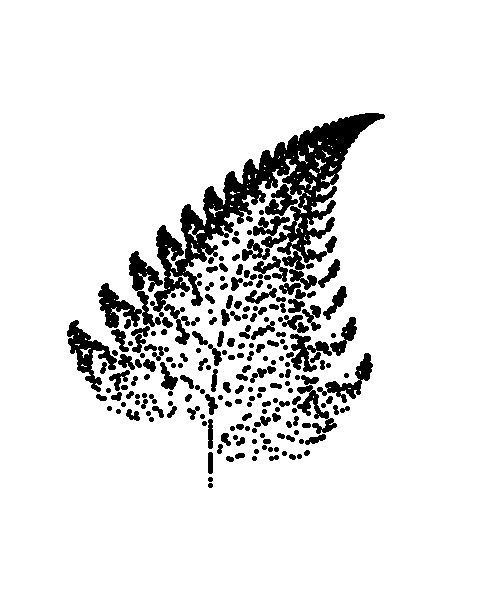1000 , 2000, 3000 Iteration

Share Article